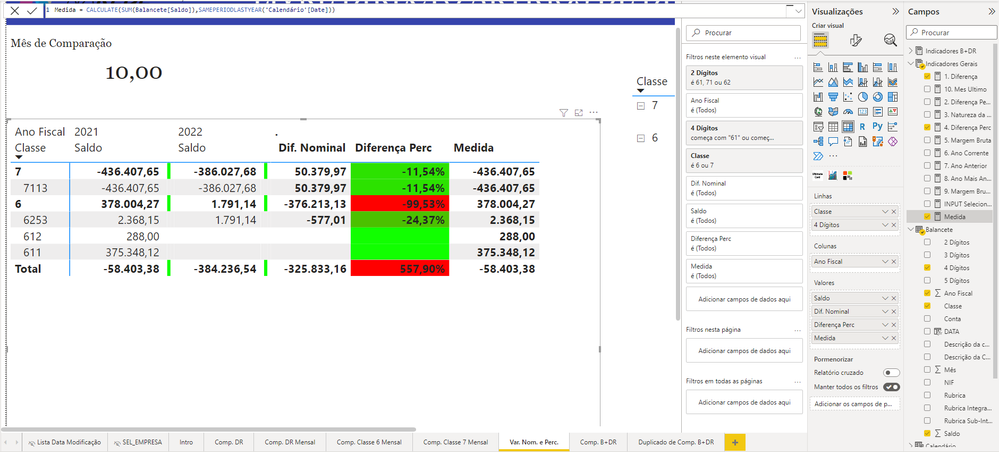cancel
Showing results for
Did you mean:New Member

## Measure Max Month from recent year + visual to filter data from that max month

Good morning,

I am having some difficulties that I don't know how to overcome.

I am building a report for accounting. My data has column YEAR and column MONTH.

I have a measure called

6. Ano Corrente = CALCULATE(MAX(BSHEET[YEAR]))

which tells me which is the most recent year that has data. So far I have data of 2022, so the result will be 2022.

• First issue is:

I want a visual CARD that tells me which is the last month of the most recent year that contains data. For that, I created a measure with the following DAX code

10. Mes Ultimo = CALCULATE(MAX(BSHEET[MONTH]), FILTER('BSHEET', 'BSHEET'[YEAR]=[6. Ano Corrente]))

which seemed the most logical but it is not working.

To give you an example, I have data of all months from previous years, but for the year 2022 I only have data from 9 months. So, the result of this measure should be 9. The result of the formula I have is giving me 12.

• Second issue is:

I have a visual matrix that shows me data from some accounts, including balance for the most recent year (2022) and the 3 previous years. This visual matrix is based on a table that is on powerquery, not on measures.

The problem is that it is showing me all data from every year. For 2019, 2020 and 2021, it is showing me data from the 12 months, but in 2022 it is showing me data from 9 months, which is what I have. But I should not compare the most recent year with previous years since the most recent year is not finished. In other words, I should not compare year 2021 that already had 12 months of data with 2022 that only has 9 months of data. It's not comparable because it is not in the same timeframe.

So I want that the measure above to filter this table and show me the data each year had from these accounts up until the last month of the most recent year that contains data.

For example, if the result of the measure above is 9, then the matrix visual has to present data from all years (2019, 2020, 2021 and 2022) only from month 1 to month 9.

Thank you very much for any help provided.

1 ACCEPTED SOLUTIONResolver I

For 1st issue, try this
10. Mes Ultimo =
var year_max = CALCULATE(MAX(BSHEET[YEAR]))

return CALCULATE(MAX(BSHEET[MONTH]), FILTER('BSHEET', 'BSHEET'[YEAR]=year_max)
For 2nd issue, you should try (same period last year) function, that will take the data for same amount of months for previous years too.
If my reply helps you then do give a thumbs up.

5 REPLIES 5Resolver I

https://learn.microsoft.com/en-us/dax/sameperiodlastyear-function-dax

Do check out above refrenced link to better understand that function.New Member

Thank you very much for your help @Nofil098.

After some research, I'm still not able to replicate the desired result.

Since last month of 2022 is month 10 (as it shows at the card at the top, using the DAX formula you provided), I want that columns before 2022 (in this case only 2021) presents the balance from month 1 to month 10 (the result of the measure [10. Mes Ultimo]) for those years.

Because the way it is now I am watching data until month 10 in 2022 and month 12 in 2021, so the data is not comparable.

Using manual filter I can do it, just drag column Month and filter what's before month 10, but I would have to do it everytime new data comes in and I want that to be automatic.Thank you in advance for the help provided.Resolver I

For 1st issue, try this
10. Mes Ultimo =
var year_max = CALCULATE(MAX(BSHEET[YEAR]))

return CALCULATE(MAX(BSHEET[MONTH]), FILTER('BSHEET', 'BSHEET'[YEAR]=year_max)
For 2nd issue, you should try (same period last year) function, that will take the data for same amount of months for previous years too.
If my reply helps you then do give a thumbs up.Frequent Visitor

Hello, I tried this function, but was unable to properly execute this. Can you assist with what I'm doing wrong?

MaxNoteMonth1 = CALCULATE(Max( 'Complete Data'[Submission Date].[Year]))
CALCULATE(Max( 'Complete Data'[Submission Date].[Month]),FILTER('Complete Data','Complete Data'[Submission Date].[Year]=2023))New Member

First issue is solved, thank you very much. Don't know why it's necessary to define that variable for this to work though.

Regarding the second issue, what do you mean try same period last year function?